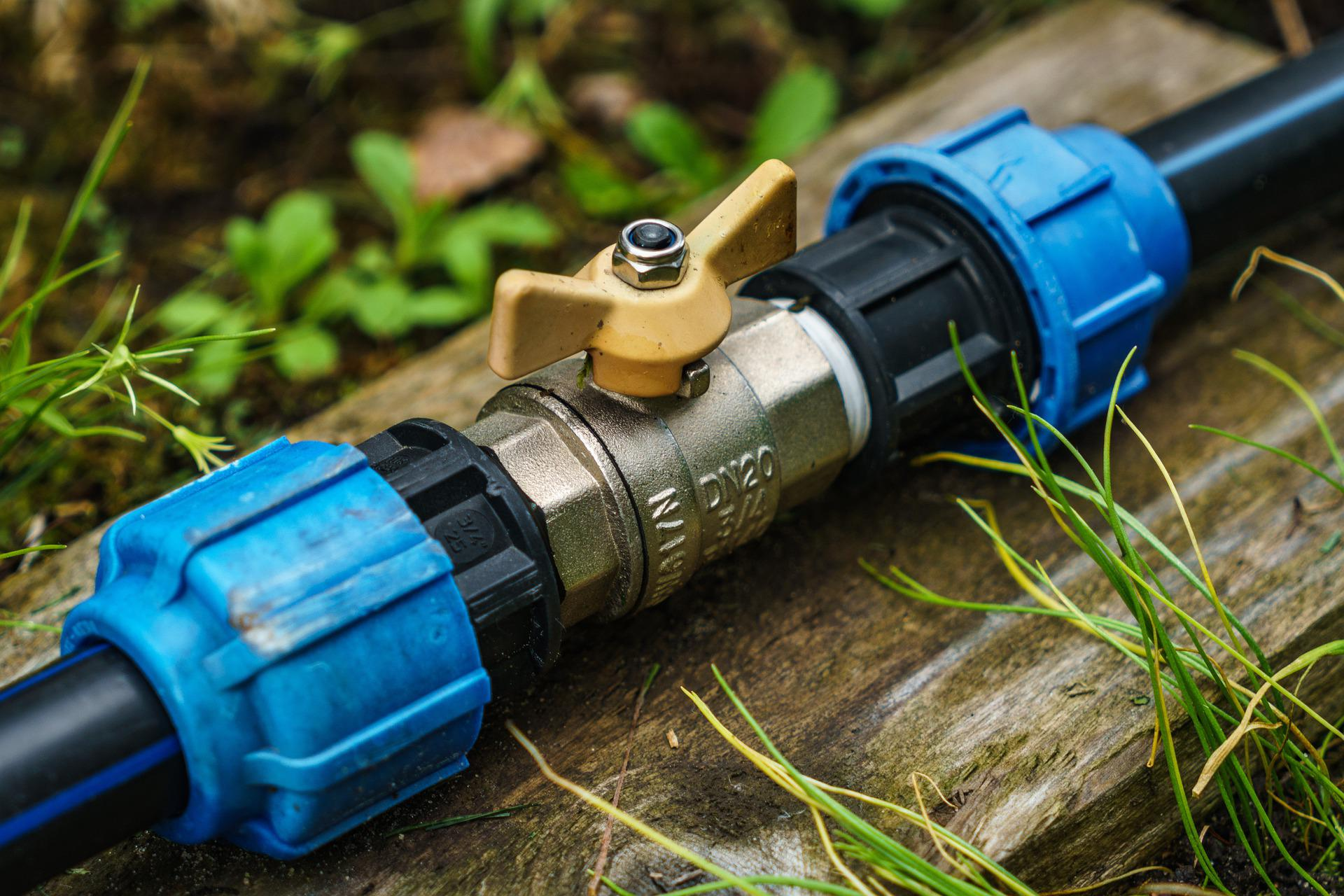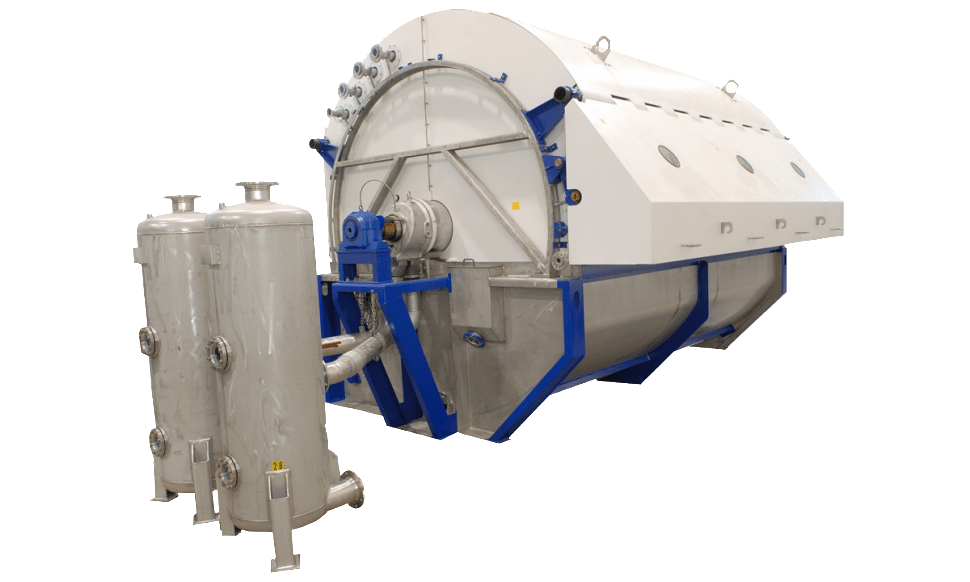## How to Check if a Horizontal Pipe Carrying Liquid is Full

In this post I want to share how to check if a horizontal pipe carrying liquid is full or partially full. We can use the following criteria to check:

• The pipe is full if: Q/d5 ≥ 10.2
• The pipe is partially full if: Q/d5 < 10.2

For partially full pipe, we need to do partially full flow analysis as follows.

x = ln (Q/d2.5)

Then we calculate height of liquid in the pipe using the following equation:

H/D = 0.446 + 0.272x + 0.0397x2 – 0.0153x3 – 0.003575x4 Read More## Basic Filtration Equation

Let’s learn the basic filtration equation which may be useful in sizing and evaluating many types of filtration unit. Disclaimer, this is also the first time in my working life learning about filtration equation, so if you find any mistakes, please feel free to comment below.

There are two terms in basic filtration equation. The first is, if the filtration occurs at constant pressure. And the second is, if the filtration occurs at constant flow rate. If the filtration occurs at constant pressure, then the flow rate will progressively diminished because the filter bed is steadily growing in thickness. Whereas, if the filtration occurs at constant flow rate, then the pressure must be gradually increased.